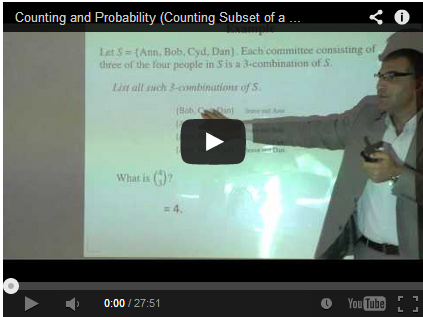### Jarrar: Discrete Mathematics Course#### Discrete Mathematics Course

 Course Outline The goal of this course is to introduce students to ideas and techniques from discrete mathematics that are widely used in computer science. Ultimately, students are expected to understand and use (abstract) discrete structures that are the backbones of computer science. In particular, this class is meant to introduce logic, proofs, sets, functions, counting and relations with an emphasis on applications in computer science.

#### By: Mustafa Jarrar